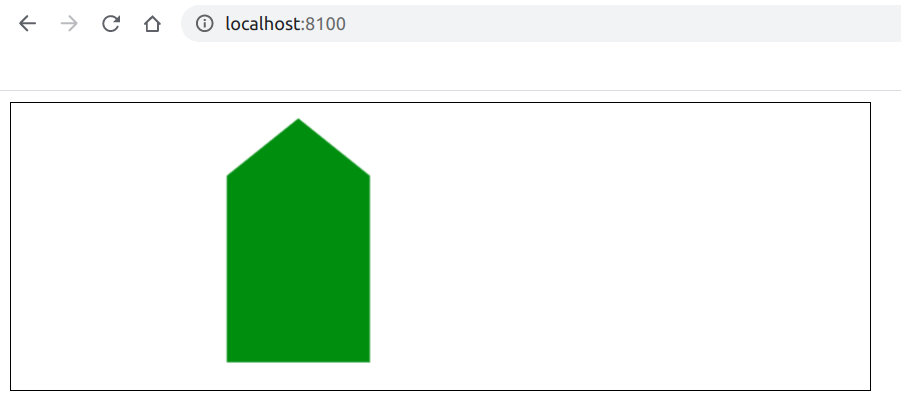Open In App

# How to create a canvas polygon using Fabric.js ?

In this article, we are going to see how to create a canvas polygon using FabricJS. The canvas means the polygon is movable and can be stretched according to requirements. Further, the polygon can be customized when it comes to the initial fill color and its coordinates.

Approach: To make it possible we are going to use a JavaScript library called FabricJS. After importing the library, we will create a canvas block in the body tag which will contain our polygon. After this, we will initialize instances of Canvas and Polygon provided by FabricJS and render the Polygon instance on the Canvas instance as given in the example below.

Syntax:

` fabric.Polygon(points, options); `

Parameters: This function accepts two parameters as mentioned above and described below:

• points: It specifies the starting and ending coordinates for all the points of the polygon.
• options: It specifies the additional options to be applied.

Example: This example uses FabricJS to create a simple editable canvas polygon.

## html

 ```<``html``>``<``head``>``    ``<``title``>``        ``How to create a canvas polygon using Fabric.js?``    ```` ` `    ````    ``<``script` `src``=``"https://cdnjs.cloudflare.com/ajax/libs/fabric.js/3.6.2/fabric.min.js"``>``    `````` ` `<``body``>``    ``<``canvas` `id``=``"canvas"``            ``width``=``"600"``            ``height``=``"200"``            ``style``=``"border:1px solid #000000;"``>``    ```` ` `    ``<``script``>`` ` `        ``// Initiate a Canvas instance``        ``var canvas = new fabric.Canvas("canvas");`` ` `        ``// Initiate a polygon instance``        ``var polygon = new fabric.Polygon([``        ``{ x: 200, y: 10 },``        ``{ x: 250, y: 50 },``        ``{ x: 250, y: 180},``        ``{ x: 150, y: 180},``        ``{ x: 150, y: 50 }], {``            ``fill: 'green'``        ``});`` ` `        ``// Render the polygon in canvas``        ``canvas.add(polygon);``    ```````

Output: